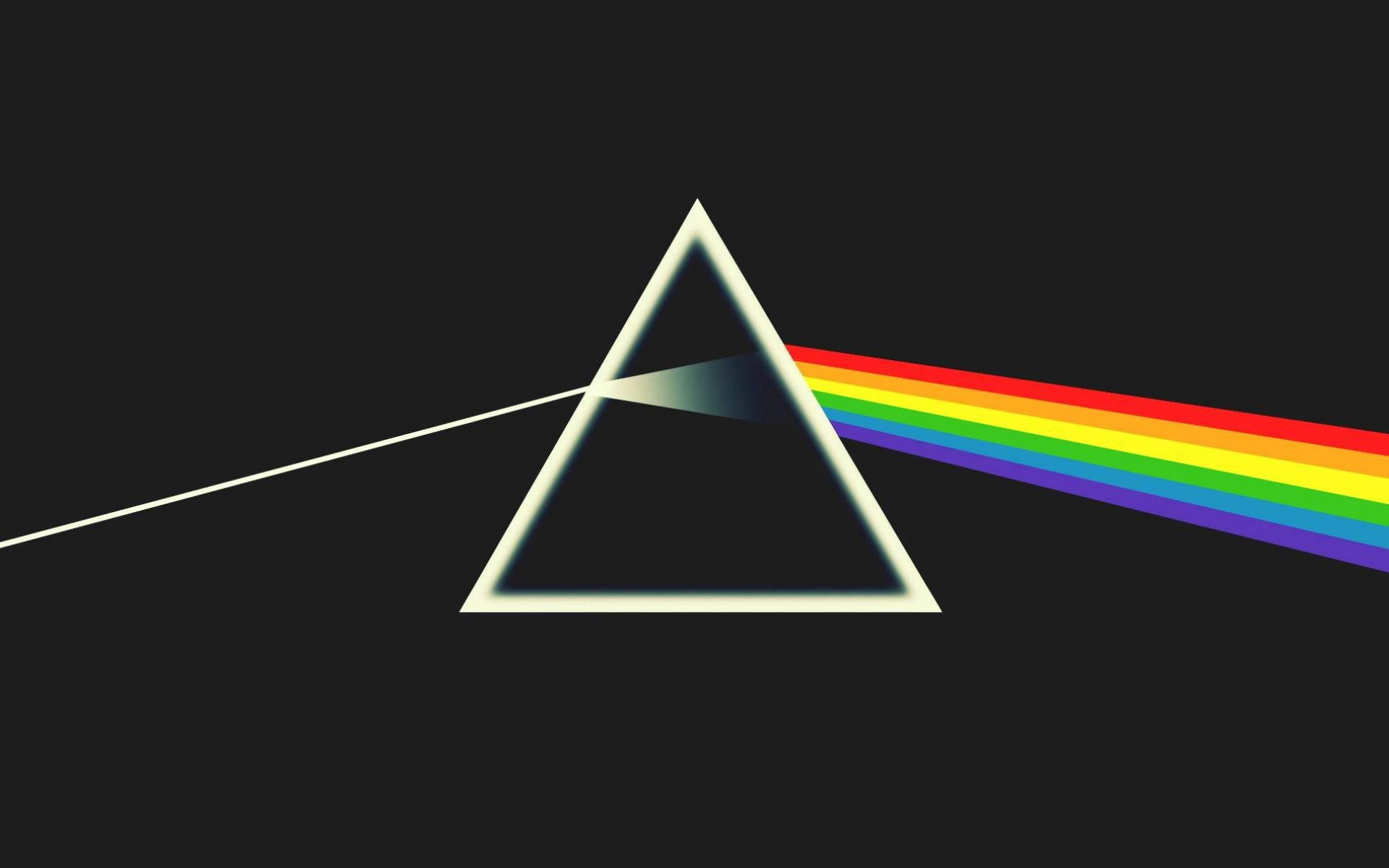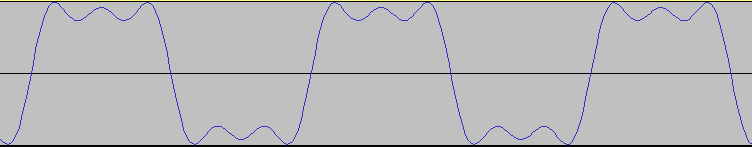# Audio From Scratch With Go: Harmonics

So far, we have seen how we can generate pure signals such as sine waves, square waves and triangle waves. These are handy signals for debugging and easy to generate, but in the real world instruments don’t generate such pure signals. For example, when plucking a guitar string it will vibrate along mutliple frequencies. These different vibrations are called the ‘harmonics’, which consist of the ‘fundamental’ frequency + overtone frequencies. So the final sound that we hear is a combination of waves vibrating along these different frequencies.

A useful way for imagining this is to draw the analogy with light. When you shine light through a prism, it will decompose into the different colours (wavelengths) that make up the light. Similarly, you could imagine a prism through which we send the sound wave to decompose it into the wavelengths making up this particular sound.(An image of a prism you’ll recognize from either physics classes or Pink Floyd)

The question then becomes, given that real instruments combine waves of different frequencies, how can we generate these programatically?

The way we will generate these waves is by applying Fourier addition. Just as in the previous post, we will store the generated series in a table which we can oscillate through with the `LookupOscillator`. At the end we will use the same `normalize` function we wrote earlier to normalize the amplitudes for the lookup table.

 `````` 1 2 3 4 5 6 7 8 9 10 11 12 13 14 15 16 `````` ``````func FourierTable(nharms int, amps []float64, length int, phase float64) []float64 { table := make([]float64, length+2) phase *= tau for i := 0; i < nharms; i++ { for n := 0; n < len(table); n++ { amp := 1.0 if i < len(amps) { amp = amps[i] } angle := float64(i+1) * (float64(n) * tau / float64(length)) table[n] += (amp * math.Cos(angle+phase)) } } return normalize(table) } ``````

In this function, we pass `nharms` to determine how many harmonics we want to generate, we pass a `[]float64` to set a different amplitude for the harmonics (or default to 1.0 if none is passed). Furthermore we will speicfy a length for the table, as well as a starting phase which is essentially an offset for the wave.

The basic idea is that for each harmonic we iterate through the entire table and increment the wave as `table[n]` with the current amplitude of the wave for that harmonic. Each subsequent harmonic oscillates at `N * fundamental` frequency so that we get (`harmonics = {1F, 2F ... NF}`), where the fundamental is “1F”.

We obtain this by changing the angle which we’ll pass to `math.Cos(..)` depending on which harmonic we are generating.

 ``````1 2 3 4 `````` `````` // Adjust angle for harmonic 'i' -> move N times forward in the wave angle := float64(i+1) * (float64(n) * tau / float64(length)) // Add wave amplitude for harmonic 'i' to existing wave (table[n]) table[n] += (amp * math.Cos(angle+phase)) ``````

## Generating waves with harmonics

Now that we have the Fourier addition in place, we still have to generate different waveforms using this generator. We can change how an harmonic looks like by passing a slice of `float64` to the `FourierTable` function, this is crucial in generating the type of harmonics we want for our wave.

For example, to generate a Square, Triangle and Saw wavetable with N harmonics:

 `````` 1 2 3 4 5 6 7 8 9 10 11 12 13 14 15 16 17 18 19 20 21 22 23 `````` ``````func SquareTable(nharms, length int) []float64 { amps := make([]float64, nharms) for i := 0; i < len(amps); i += 2 { amps[i] = 1.0 / float64(i+1) } return FourierTable(nharms, amps, length, -0.25) } func SawTable(nharms, length int) []float64 { amps := make([]float64, nharms) for i := 0; i < len(amps); i++ { amps[i] = 1.0 / float64(i+1) } return FourierTable(nharms, amps, length, -0.25) } func TriangleTable(nharms, length int) []float64 { amps := make([]float64, nharms) for i := 0; i < nharms; i += 2 { amps[i] = 1.0 / (float64(i+1) * float64(i+1)) } return FourierTable(nharms, amps, length, 0) } ``````

To see what this looks and sounds like, we can create a small test program to generate such waves. Such a program is included in the GoAudio examples

To run this program to generate a square wave, with 6 harmonics and a max amplitude of 0.8, at frequency 440 during 4 seconds we would use this command:

``````go run main.go -d 4 -s square -a 0.8 -h 6 -f 440 -o output.wav
``````

The output will look like this in audacityListen to what it sounds like

We now have the basics to start making some simple tunes with GoAudio, which will be the topic of the next post.

If you liked this and want to know when I write new posts, the best way to keep up to date is by following me on twitter.